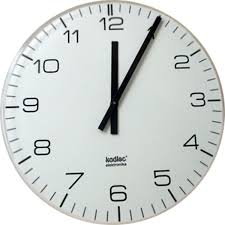Jack had 4 hours of school.

He spent 45 minutes in the library and 1/2 hour on a science lecture and had a lunch break of 25 minutes.

How much time is left for the school to get over? (Write the answer as a fraction.)

x =  7/3 = 2 13 h

### Step-by-step explanation:Did you find an error or inaccuracy? Feel free to write us. Thank you!

Tips for related online calculators
Need help calculating sum, simplifying, or multiplying fractions? Try our fraction calculator.
Do you want to convert time units like minutes to seconds?